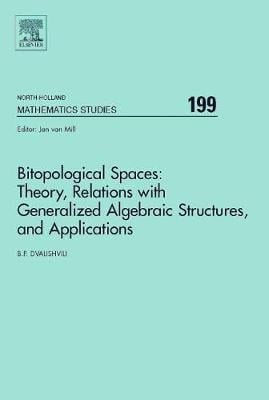•# Bitopological Spaces: Theory, Relations with Generalized Algebraic Structures and Applications: Volume 199 - North-Holland Mathematics Studies (Hardback)

(author)
£145.00
Hardback 422 Pages / Published: 20/01/2005
• We can order this

Usually dispatched within 3 weeks

This monograph is the first and an initial introduction to the theory of bitopological spaces and its applications. In particular, different families of subsets of bitopological spaces are introduced and various relations between two topologies are analyzed on one and the same set; the theory of dimension of bitopological spaces and the theory of Baire bitopological spaces are constructed, and various classes of mappings of bitopological spaces are studied. The previously known results as well the results obtained in this monograph are applied in analysis, potential theory, general topology, and theory of ordered topological spaces. Moreover, a high level of modern knowledge of bitopological spaces theory has made it possible to introduce and study algebra of new type, the corresponding representation of which brings one to the special class of bitopological spaces. It is beyond any doubt that in the nearest future the areas of essential applications will be the theories of linear topological spaces and topological groups, algebraic and differential topologies, the homotopy theory, not to mention other fundamental areas of modern mathematics such as geometry, mathematical logic, the probability theory and many other areas, including those of applied nature. Key Features: - First monograph is "Generalized Lattices"

Publisher: Elsevier Science & Technology
ISBN: 9780444517937
Number of pages: 422
Weight: 910 g
Dimensions: 240 x 165 x 23 mm